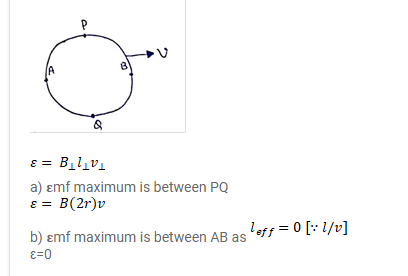# A copper circular-ring of radius r translates in its plane with

Question:

A copper circular-ring of radius $r$ translates in its plane with a constant velocity $v$. A uniform magnetic field B exists in the space in a direction perpendicular to the plane of the ring. Consider different pairs of diametrically opposite points on the ring.

(a) Between which pair of points is the emf maximum? What is the value of this maximum emf?

(b) Between which pair of points is the emf minimum? What is the value of this minimum emf?

Solution: